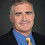## Wednesday, July 19, 2023

### More on Pseudodeterminism

Back in May I posted about a paper that finds primes pseudodeterministically and Quanta magazine recently published a story on the result. Oddly enough the paper really isn't about primes at all.

Consider any set A such that

1. A is easy to compute, i.e., A is in P.
2. A is very large, that is there is a c such that for all n, the number of strings of length n is at least 2n/nc. If you throw a polynomial number of darts you are very likely to hit A.
Chen et al show that for any such A, there is a probabilistic polynomial time Turing machine M such that for infinitely many x in A, M on input 1|x| will output x with high probability.

The set of primes has property 1 by Agrawal, Kayal and Saxena and property 2 by the prime number theorem. Why focus the paper on primes though? Because deterministic prime number finding is a long-standing open problem and there aren't many other natural problems that have properties 1 and 2 where we don't know easy deterministic algorithms to find examples.

With the general result, we can think about oracle results. It's pretty easy to create an A, such that A has property 2 and no deterministic polynomial-time algorithm with oracle access to A can find an element of length n on input 1n for infinitely many n. Since A is always in PA we get property 1 for free. That would suggest to push the result to finding primes deterministically would require using properties of the primes beyond being large and easy.

Maybe not. Rahul Santhanam, one of the authors, tells me their proof doesn't relativize, though whether the result relativizes is an open question, i.e. whether there is an A fulfilling property 2 such that any pseudodeterministic algorithm with oracle access to A will fail to find more than a finite number of elements of A. It does seem hard to construct this oracle, even if we just want the pseudodeterministic algorithms to fail for infinitely many n.

Nevertheless it seems unlikely you'd be able to use these techniques to prove a deterministic algorithm for all large easy A. If you want to find primes deterministically you'll probably need some to prove some new number theory.

1.Psuedodeterminism -> Pseudodeterminism

1.2.I am puzzled by the fact that one needs A∈P. I would have guessed that A∈ZPP (or at least A∈RP or coRP) would be enough. Is there an easy explanation for this?

1.If A is in RP via a machine M consider the set B=(x,r) such that M(x) accepts using random coins r. B would have properties 1 and 2 so we could find a (x,r) pseudodeterministically and drop the r to find an x in A. However the proof that Primes are in RP was more complicated than the proof that Primes are in P.

3.Also, the fact that it does not really depend on numbers being prime reminds me of the proof of Green-Tao Theorem: The proof uses a generalized Szemerédi's theorem, about arithmetic progressions for dense enough sets (for some definition of "dense enough"), and the fact that prime numbers are dense enough in the required sense. Of course, proving the density of primes in the required sense is hard while it is easy and well-known for the new algorithm, but at least from a high-level, this is the same proof idea. (And many other results about prime numbers have this kind of proof.)

4.Right now it is unclear how to prove the same result for A in BPP or ZPP. The construction has the unusual feature (in derandomization results) that the *code* of the algorithm computing A affects the canonical strings in A generated by the pseudodeterministic algorithm. It is unclear how to maintain the pseudodeterministic property if we have a randomized algorithm for A.

5.This seems to be a typo: "for infinitely many x, M on input 1^|x| will output x with high probability." Presumably you mean that for infinitely many x, M on input 1^|x| outputs an element of A and with high probability, it is the same element of A (high probability over the choice of random coins used by M(1^|x|)).

1.Right. I added "x in A" which should fix this.

2.I also misread the original statement so my comment is not very clear. The main typo was, as you say, simply leaving out "x in A" (and it doesn't seem like a serious typo since x being in A is probably already obvious from the context). Sorry for the confusion.

6.Thanks. Fixed.